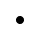Mobile3May 23, 2022

# The Introduction To Artificial Intelligence Algorithms #1 - HillClimbing

In this series of articles, we will gradually get acquainted with several algorithms of artificial intelligence. We will look at how such "intelligent" algorithms work and where exactly we can use them as programmers. I will help you along the way from optimization algorithms, through search algorithms to neural networks and machine learning.

Personally, I use this knowledge in shoe programming on the codingame.com platform and with some basic principles I share it with the students of the technical high school in Nová Dubnica, where I teach several programming subjects.

Therefore, this series will also be conducted in such a way that everyone who does not like letters in mathematics can understand them.

# Optimization algorithms

Several artificial intelligence algorithms consist of creating a bad solution and its subsequent tuning / optimization. We can consider such a bad solution, for example, a trip from Bratislava to Košice through Prague, or a coloring book painted with the wrong colors. For example, in the case of artificial intelligence in computer games, we could talk about accidental keystrokes. Optimization algorithms are also used for learning neural networks.

With such problems, we as humans know relatively quickly to find a solution that is approaching the ideal. However, the ideal is extremely difficult to achieve, especially when our solutions are affected by external influences. For example, just add one player to a single-player game ...

All optimization algorithms work as follows:

1. Create n random solutions (1 vector, population of n individuals, 1 number, etc.)
2. Until you are satisfied with the solution / your time runs out
a. slightly modify these solutions
b. if you find a better solution save it

## HillClimbing algorithm

Imagine a mobile game in which we use a finger to control the direction and strength of a golf ball on a mini golf course. Our task is to hit the hole for as few shots as possible. Strength and direction are the only 2 parameters we can influence. How do we teach our artificial intelligence to hit the ball correctly?

It is not so difficult to find a good solution to this small problem. But let's do the procedure I described above. We will create a random solution and try to modify it slowly.

1. Let's create 1 random solution, e.g. solution = {force: 50, direction: 60}
2. Infinitely
a. we will create 4 new solutions such where new_solutions = [

{force + 1, direction},

{force - 1, direction},

{force, direction + 1},

{force, direction -1}]
]

b. is any solution from the new solution better than the solution?
i. no - end the cycle

ii. yes - solution = new_solutions [best]

Great! We call this algorithm a climbing algorithm. Let's see what the first iteration of this algorithm looks like:

The middle arrow shows the force and direction of the strike without any adjustment of the parameters. The other arrows show all four neighbours, which I can get by minor parameter adjustment.

All we have to do is think about how we will evaluate the new solution as better. We will not program the whole game, so we will just set the correct result for e.g. 92 resp. 15 for strength and direction. The cost of the solution will be the sum of the differences between the expected and current direction and strength. Our solution in the form of a climbing algorithm would look like this (Kotlin):

This solution would work great for a playing area without any obstructions and if the initial direction of the swing is within 45 degrees of the target position. This is because hillclimbing can't get out of local optima in any case. For an angle greater than 45 degrees, the algorithm would evaluate the optimal solution to be the one where the strike force = 0 so that we do not move away from the target.

If you would like to try the behavior of the HillClimb algorithm on an example similar to the one I presented, you can try it with this gist. It incorporates an extremely simple simulation of the ball's movement and at the same time implements the HillClimb algorithm and a visual representation of a minigolf course.

## Stay tuned

Although we didn't rely much on theory, we were able to show how one of the most basic optimization algorithms works. HillClimbing searches the nearest neighborhood and always chooses only the most friendly solution from this neighborhood. If it finds no more beautiful solution, it will end the search.

We will show you Taboo Search next time and we will talk a bit about what features these algorithms actually have.

### Self-study

I recommend MIT lectures on artificial intelligence algorithms